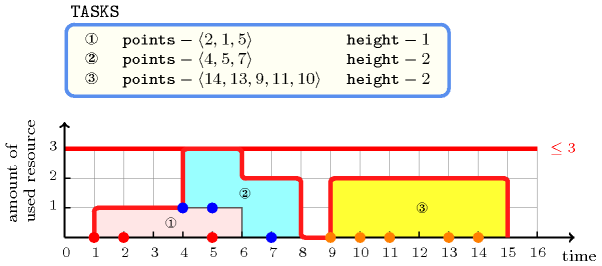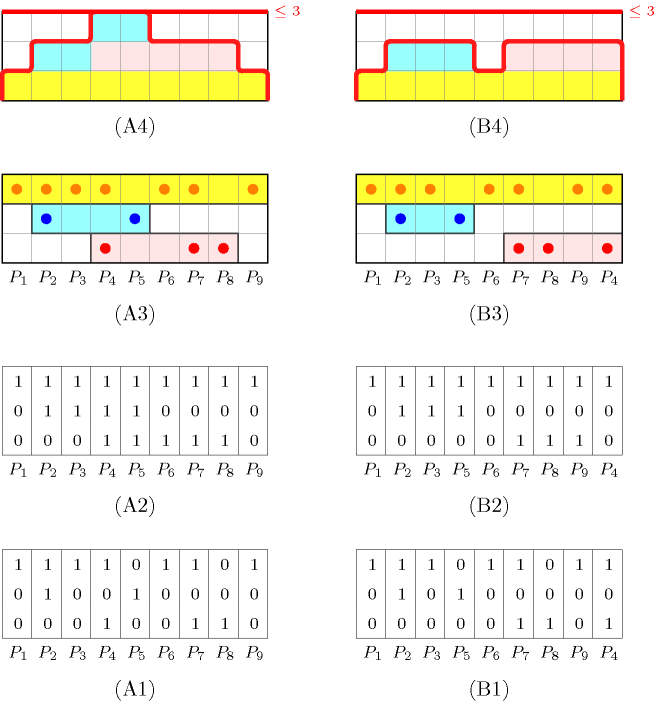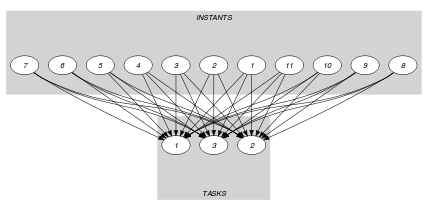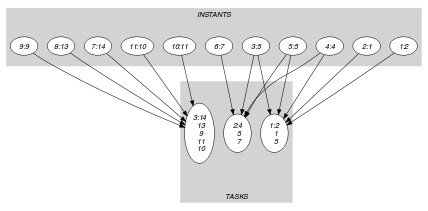## 5.97. cumulative_convex

Origin
Constraint

$\mathrm{𝚌𝚞𝚖𝚞𝚕𝚊𝚝𝚒𝚟𝚎}_\mathrm{𝚌𝚘𝚗𝚟𝚎𝚡}\left(\mathrm{𝚃𝙰𝚂𝙺𝚂},\mathrm{𝙻𝙸𝙼𝙸𝚃}\right)$

Type
 $\mathrm{𝙿𝙾𝙸𝙽𝚃𝚂}$ $\mathrm{𝚌𝚘𝚕𝚕𝚎𝚌𝚝𝚒𝚘𝚗}\left(\mathrm{𝚟𝚊𝚛}-\mathrm{𝚍𝚟𝚊𝚛}\right)$
Arguments
 $\mathrm{𝚃𝙰𝚂𝙺𝚂}$ $\mathrm{𝚌𝚘𝚕𝚕𝚎𝚌𝚝𝚒𝚘𝚗}\left(\mathrm{𝚙𝚘𝚒𝚗𝚝𝚜}-\mathrm{𝙿𝙾𝙸𝙽𝚃𝚂},\mathrm{𝚑𝚎𝚒𝚐𝚑𝚝}-\mathrm{𝚍𝚟𝚊𝚛}\right)$ $\mathrm{𝙻𝙸𝙼𝙸𝚃}$ $\mathrm{𝚒𝚗𝚝}$
Restrictions
 $\mathrm{𝚛𝚎𝚚𝚞𝚒𝚛𝚎𝚍}$$\left(\mathrm{𝙿𝙾𝙸𝙽𝚃𝚂},\mathrm{𝚟𝚊𝚛}\right)$ $|\mathrm{𝙿𝙾𝙸𝙽𝚃𝚂}|>0$ $\mathrm{𝚛𝚎𝚚𝚞𝚒𝚛𝚎𝚍}$$\left(\mathrm{𝚃𝙰𝚂𝙺𝚂},\left[\mathrm{𝚙𝚘𝚒𝚗𝚝𝚜},\mathrm{𝚑𝚎𝚒𝚐𝚑𝚝}\right]\right)$ $\mathrm{𝚃𝙰𝚂𝙺𝚂}.\mathrm{𝚑𝚎𝚒𝚐𝚑𝚝}\ge 0$ $\mathrm{𝙻𝙸𝙼𝙸𝚃}\ge 0$
Purpose

Cumulative scheduling constraint or scheduling under resource constraints. Consider a set $𝒯$ of tasks described by the $\mathrm{𝚃𝙰𝚂𝙺𝚂}$ collection where each task is defined by:

• A set of distinct points depicting the time interval where the task is actually running: the smallest and largest coordinates of these points respectively give the first and last instant of that time interval.

• A height that depicts the resource consumption used by the task from its first instant to its last instant.

The $\mathrm{𝚌𝚞𝚖𝚞𝚕𝚊𝚝𝚒𝚟𝚎}_\mathrm{𝚌𝚘𝚗𝚟𝚎𝚡}$ constraint enforces that, at each point in time, the cumulated height of the set of tasks that overlap that point, does not exceed a given limit. A task overlaps a point $i$ if and only if (1) its origin is less than or equal to $i$, and (2) its end is strictly greater than $i$.

Example
$\left(\begin{array}{c}〈\begin{array}{cc}\mathrm{𝚙𝚘𝚒𝚗𝚝𝚜}-〈2,1,5〉\hfill & \mathrm{𝚑𝚎𝚒𝚐𝚑𝚝}-1,\hfill \\ \mathrm{𝚙𝚘𝚒𝚗𝚝𝚜}-〈4,5,7〉\hfill & \mathrm{𝚑𝚎𝚒𝚐𝚑𝚝}-2,\hfill \\ \mathrm{𝚙𝚘𝚒𝚗𝚝𝚜}-〈14,13,9,11,10〉\hfill & \mathrm{𝚑𝚎𝚒𝚐𝚑𝚝}-2\hfill \end{array}〉,3\hfill \end{array}\right)$

Figure 5.97.1 shows the cumulated profile associated with the example. To each set of points defining a task corresponds a rectangle. The height of each rectangle represents the resource consumption of the associated task. The $\mathrm{𝚌𝚞𝚖𝚞𝚕𝚊𝚝𝚒𝚟𝚎}_\mathrm{𝚌𝚘𝚗𝚟𝚎𝚡}$ constraint holds since at each point in time we do not have a cumulated resource consumption strictly greater than the upper limit 3 enforced by the last argument of the $\mathrm{𝚌𝚞𝚖𝚞𝚕𝚊𝚝𝚒𝚟𝚎}_\mathrm{𝚌𝚘𝚗𝚟𝚎𝚡}$ constraint.

##### Figure 5.97.1. Points defining the three tasks of the Example slot and corresponding resource consumption profile (note that the vertical position of a task does not really matter but is only used for displaying the contribution of a task to the resource consumption profile)Typical
 $|\mathrm{𝚃𝙰𝚂𝙺𝚂}|>1$ $\mathrm{𝚃𝙰𝚂𝙺𝚂}.\mathrm{𝚑𝚎𝚒𝚐𝚑𝚝}>0$ $\mathrm{𝙻𝙸𝙼𝙸𝚃}<$$\mathrm{𝚜𝚞𝚖}$$\left(\mathrm{𝚃𝙰𝚂𝙺𝚂}.\mathrm{𝚑𝚎𝚒𝚐𝚑𝚝}\right)$
Symmetries
• Items of $\mathrm{𝚃𝙰𝚂𝙺𝚂}$ are permutable.

• Items of $\mathrm{𝚃𝙰𝚂𝙺𝚂}.\mathrm{𝚙𝚘𝚒𝚗𝚝𝚜}$ are permutable.

• $\mathrm{𝚃𝙰𝚂𝙺𝚂}.\mathrm{𝚑𝚎𝚒𝚐𝚑𝚝}$ can be decreased to any value $\ge 0$.

• $\mathrm{𝙻𝙸𝙼𝙸𝚃}$ can be increased.

Arg. properties

Contractible wrt. $\mathrm{𝚃𝙰𝚂𝙺𝚂}$.

Usage

A natural use of the $\mathrm{𝚌𝚞𝚖𝚞𝚕𝚊𝚝𝚒𝚟𝚎}_\mathrm{𝚌𝚘𝚗𝚟𝚎𝚡}$ constraint corresponds to problems where a task is defined as the convex hull of a set of distinct points ${P}_{1},\cdots ,{P}_{n}$ that are not initially fixed. Note that, by explicitly introducing a start $S$ and an end $E$ variables, and by using a $\mathrm{𝚖𝚒𝚗𝚒𝚖𝚞𝚖}$$\left(S,〈\mathrm{𝚟𝚊𝚛}-{P}_{1},\cdots ,\mathrm{𝚟𝚊𝚛}-{P}_{n}〉\right)$ and a $\mathrm{𝚖𝚊𝚡𝚒𝚖𝚞𝚖}$$\left(E,〈\mathrm{𝚟𝚊𝚛}-{P}_{1},\cdots ,\mathrm{𝚟𝚊𝚛}-{P}_{n}〉\right)$ constraints, one could replace the $\mathrm{𝚌𝚞𝚖𝚞𝚕𝚊𝚝𝚒𝚟𝚎}_\mathrm{𝚌𝚘𝚗𝚟𝚎𝚡}$ constraint by a $\mathrm{𝚌𝚞𝚖𝚞𝚕𝚊𝚝𝚒𝚟𝚎}$ constraint. However this hinders propagation.

As a concrete example of use of the $\mathrm{𝚌𝚞𝚖𝚞𝚕𝚊𝚝𝚒𝚟𝚎}_\mathrm{𝚌𝚘𝚗𝚟𝚎𝚡}$ constraint we present a constraint model for a well-known pattern-sequencing problem [FinkVoss99] (also known to be equivalent to the graph path-width [LinharesYanasse02] problem) that is based on a single $\mathrm{𝚌𝚞𝚖𝚞𝚕𝚊𝚝𝚒𝚟𝚎}_\mathrm{𝚌𝚘𝚗𝚟𝚎𝚡}$ constraint. The pattern sequencing problem can be described as follows: Given a 0-1 matrix in which each column $j$ $\left(1\le j\le p\right)$ corresponds to a product required by the customers and each row $i$ $\left(1\le i\le c\right)$ corresponds to the order of a particular customer (The entry ${c}_{ij}$ is equal to 1 if and only if customer $i$ has ordered some quantity of product $j$.), the objective is to find a permutation of the products such that the maximum number of open orders at any point in the sequence is minimised. Order $i$ is open at point $k$ in the production sequence if there is a product required in order $i$ that appears at or before position $k$ in the sequence and also a product that appears at or after position $k$ in the sequence.

##### Figure 5.97.2. An input matrix for the pattern sequencing problem (A1), its corresponding cumulated matrix (A2), a view in term of tasks (A3) and the corresponding cumulative profile (A4); a second matrix (B1) where column 4 of (A1) is put at the rightmost position.Before giving the constraint model, let us first provide an instance of the pattern-sequencing problem. Consider the matrix ${ℳ}_{1}$ depicted by part (A1) of Fig. 5.97.2. Part (A2) gives its corresponding cumulated matrix ${ℳ}_{2}$ obtained by setting to 1 each 0 of ${ℳ}_{1}$ that is both preceded and followed by a 1. Part (A3) depicts the corresponding solution in term of the $\mathrm{𝚌𝚞𝚖𝚞𝚕𝚊𝚝𝚒𝚟𝚎}_\mathrm{𝚌𝚘𝚗𝚟𝚎𝚡}$ constraint: to each row of the matrix ${ℳ}_{1}$ corresponds a task defined as the convex hull of the different 1 located on that row. Finally part (A4) gives the cumulated profile associated with part (A3), namely the number of 1 in each column of ${ℳ}_{2}$. The cost 3 of this solution is equal to the maximum number of 1 in the columns of the cumulated matrix ${ℳ}_{2}$. As shown by parts (B1-B4), we can get a lower cost of 2 by pushing the fourth column to the rightmost position.

The idea of the model is to associate to each row (i.e., customer) $i$ of the cumulated matrix a stack task that starts at the first 1 on row $i$ and ends at the last 1 of row $i$ (i.e., the task corresponds to the convex hull of the different 1 located on row $i$). Then the cost of a solution is simply the maximum height on the corresponding cumulated profile.

For each column $j$ of the 0-1 matrix initially given there is a variable ${V}_{j}$ ranging from 1 to the number of columns $p$. The value of ${V}_{j}$ gives the position of column $j$ in a solution. We put all the stack tasks in a $\mathrm{𝚌𝚞𝚖𝚞𝚕𝚊𝚝𝚒𝚟𝚎}_\mathrm{𝚌𝚘𝚗𝚟𝚎𝚡}$ constraint, telling that each stack task uses one unit of the resource during all it execution. Since we want to have the same model for different limits on the maximum number of open stacks, and since all variables ${V}_{1},{V}_{2},\cdots ,{V}_{p}$ have to be distinct, we have an extra dummy task characterised as the convex hull of ${V}_{1},{V}_{2},\cdots ,{V}_{p}$. This extra dummy task has a height $H$ that has to be maximised. For the matrix depicted by (A1) of Fig. 5.97.2 we pass to the $\mathrm{𝚌𝚞𝚖𝚞𝚕𝚊𝚝𝚒𝚟𝚎}_\mathrm{𝚌𝚘𝚗𝚟𝚎𝚡}$ constraint the following collection of tasks:

$〈\begin{array}{cc}\mathrm{𝚙𝚘𝚒𝚗𝚝𝚜}-〈{P}_{1},{P}_{2},{P}_{3},{P}_{4},{P}_{6},{P}_{7},{P}_{9}〉\hfill & \mathrm{𝚑𝚎𝚒𝚐𝚑𝚝}-1,\hfill \\ \mathrm{𝚙𝚘𝚒𝚗𝚝𝚜}-〈{P}_{2},{P}_{5}〉\hfill & \mathrm{𝚑𝚎𝚒𝚐𝚑𝚝}-1,\hfill \\ \mathrm{𝚙𝚘𝚒𝚗𝚝𝚜}-〈{P}_{4},{P}_{7},{P}_{8}〉\hfill & \mathrm{𝚑𝚎𝚒𝚐𝚑𝚝}-1,\hfill \\ \mathrm{𝚙𝚘𝚒𝚗𝚝𝚜}-〈{P}_{1},{P}_{2},{P}_{3},{P}_{4},{P}_{5},{P}_{6},{P}_{7},{P}_{8},{P}_{9}〉\hfill & \mathrm{𝚑𝚎𝚒𝚐𝚑𝚝}-0\hfill \end{array}〉$
Algorithm

A first natural way to handle the $\mathrm{𝚌𝚞𝚖𝚞𝚕𝚊𝚝𝚒𝚟𝚎}_\mathrm{𝚌𝚘𝚗𝚟𝚎𝚡}$ constraint is to accumulate the compulsory part [Lahrichi82] of the different tasks in a profile and to prune according to this profile. We give the main ideas for computing the compulsory part of a task and for pruning a task according to the profile of compulsory parts.

Compulsory part of a task Given a task $T$ characterised as the convex hull of a set of distinct points ${P}_{1},{P}_{2},\cdots ,{P}_{k}$ the compulsory part of $T$ corresponds to the, possibly empty, interval $\left[{s}_{T},{e}_{T}\right]$ where:

• ${s}_{T}$ is the largest value $v$ such that, when all variables ${P}_{1},{P}_{2},\cdots ,{P}_{k}$ are greater than or equal to $v$, all variables ${P}_{1},{P}_{2},\cdots ,{P}_{k}$ can still take distinct values.

• ${e}_{T}$ is the smallest value $v$ such that, when all variables ${P}_{1},{P}_{2},\cdots ,{P}_{k}$ are less than or equal to $v$, all variables ${P}_{1},{P}_{2},\cdots ,{P}_{k}$ can still take distinct values.

Pruning according to the profile of compulsory parts Given two instants $i$ and $j$ $\left(i and a task $T$ characterised as the convex hull of a set of distinct points ${P}_{1},{P}_{2},\cdots ,{P}_{k}$, assume that $T$ cannot overlap $i$ and $j$ since this would lead exceeding $\mathrm{𝙻𝙸𝙼𝙸𝚃}$, the second argument of the $\mathrm{𝚌𝚞𝚖𝚞𝚕𝚊𝚝𝚒𝚟𝚎}_\mathrm{𝚌𝚘𝚗𝚟𝚎𝚡}$ constraint. Furthermore assume that, when all variables ${P}_{1},{P}_{2},\cdots ,{P}_{k}$ are both greater than $i$ and less than $j$, all variables ${P}_{1},{P}_{2},\cdots ,{P}_{k}$ cannot take distinct values. Then all values of $\left[i+1,j-1\right]$ can be removed from variables ${P}_{1},{P}_{2},\cdots ,{P}_{k}$.

Keywords
Derived Collection
$\mathrm{𝚌𝚘𝚕}\left(\begin{array}{c}\mathrm{𝙸𝙽𝚂𝚃𝙰𝙽𝚃𝚂}-\mathrm{𝚌𝚘𝚕𝚕𝚎𝚌𝚝𝚒𝚘𝚗}\left(\mathrm{𝚒𝚗𝚜𝚝𝚊𝚗𝚝}-\mathrm{𝚍𝚟𝚊𝚛}\right),\hfill \\ \mathrm{𝚒𝚝𝚎𝚖}\left(\mathrm{𝚒𝚗𝚜𝚝𝚊𝚗𝚝}-\mathrm{𝚃𝙰𝚂𝙺𝚂}.\mathrm{𝚙𝚘𝚒𝚗𝚝𝚜}.\mathrm{𝚟𝚊𝚛}\right)\right]\hfill \end{array}\right)$
Arc input(s)

$\mathrm{𝚃𝙰𝚂𝙺𝚂}$

Arc generator
$\mathrm{𝑆𝐸𝐿𝐹}$$↦\mathrm{𝚌𝚘𝚕𝚕𝚎𝚌𝚝𝚒𝚘𝚗}\left(\mathrm{𝚝𝚊𝚜𝚔𝚜}\right)$

Arc arity
Arc constraint(s)
$\mathrm{𝚊𝚕𝚕𝚍𝚒𝚏𝚏𝚎𝚛𝚎𝚗𝚝}$$\left(\mathrm{𝚝𝚊𝚜𝚔𝚜}.\mathrm{𝚙𝚘𝚒𝚗𝚝𝚜}\right)$
Graph property(ies)
$\mathrm{𝐍𝐀𝐑𝐂}$$=|\mathrm{𝚃𝙰𝚂𝙺𝚂}|$

Arc input(s)

$\mathrm{𝙸𝙽𝚂𝚃𝙰𝙽𝚃𝚂}$ $\mathrm{𝚃𝙰𝚂𝙺𝚂}$

Arc generator
$\mathrm{𝑃𝑅𝑂𝐷𝑈𝐶𝑇}$$↦\mathrm{𝚌𝚘𝚕𝚕𝚎𝚌𝚝𝚒𝚘𝚗}\left(\mathrm{𝚒𝚗𝚜𝚝𝚊𝚗𝚝𝚜},\mathrm{𝚝𝚊𝚜𝚔𝚜}\right)$

Arc arity
Arc constraint(s)
$\mathrm{𝚋𝚎𝚝𝚠𝚎𝚎𝚗}_\mathrm{𝚖𝚒𝚗}_\mathrm{𝚖𝚊𝚡}$$\left(\mathrm{𝚒𝚗𝚜𝚝𝚊𝚗𝚝𝚜}.\mathrm{𝚒𝚗𝚜𝚝𝚊𝚗𝚝},\mathrm{𝚝𝚊𝚜𝚔𝚜}.\mathrm{𝚙𝚘𝚒𝚗𝚝𝚜}\right)$
Graph class
 $•$$\mathrm{𝙰𝙲𝚈𝙲𝙻𝙸𝙲}$ $•$$\mathrm{𝙱𝙸𝙿𝙰𝚁𝚃𝙸𝚃𝙴}$ $•$$\mathrm{𝙽𝙾}_\mathrm{𝙻𝙾𝙾𝙿}$

Sets
$\begin{array}{c}\mathrm{𝖲𝖴𝖢𝖢}↦\hfill \\ \left[\begin{array}{c}\mathrm{𝚜𝚘𝚞𝚛𝚌𝚎},\hfill \\ \mathrm{𝚟𝚊𝚛𝚒𝚊𝚋𝚕𝚎𝚜}-\mathrm{𝚌𝚘𝚕}\left(\begin{array}{c}\mathrm{𝚅𝙰𝚁𝙸𝙰𝙱𝙻𝙴𝚂}-\mathrm{𝚌𝚘𝚕𝚕𝚎𝚌𝚝𝚒𝚘𝚗}\left(\mathrm{𝚟𝚊𝚛}-\mathrm{𝚍𝚟𝚊𝚛}\right),\hfill \\ \mathrm{𝚒𝚝𝚎𝚖}\left(\mathrm{𝚟𝚊𝚛}-\mathrm{𝚃𝙰𝚂𝙺𝚂}.\mathrm{𝚑𝚎𝚒𝚐𝚑𝚝}\right)\right]\hfill \end{array}\right)\hfill \end{array}\right]\hfill \end{array}$

Constraint(s) on sets
$\mathrm{𝚜𝚞𝚖}_\mathrm{𝚌𝚝𝚛}$$\left(\mathrm{𝚟𝚊𝚛𝚒𝚊𝚋𝚕𝚎𝚜},\le ,\mathrm{𝙻𝙸𝙼𝙸𝚃}\right)$
Graph model

The first graph constraint forces for each task that the set of points defining its time interval are all distinct. The second graph constraint makes sure for each time point $t$, that the cumulated heights of the tasks that overlap $t$ does not exceed the limit of the resource.

Parts (A) and (B) of Figure 5.97.3 respectively show the initial and final graph associated with the second graph constraint of the Example slot. On the one hand, each source vertex of the final graph can be interpreted as a time point corresponding to a point used in the definitions of the different tasks. On the other hand, the successors of a source vertex correspond to those tasks that overlap a given time point. The $\mathrm{𝚌𝚞𝚖𝚞𝚕𝚊𝚝𝚒𝚟𝚎}_\mathrm{𝚌𝚘𝚗𝚟𝚎𝚡}$ constraint holds since, for each successor set $𝒮$ of the final graph, the sum of the heights of the tasks in $𝒮$ does not exceed the limit $\mathrm{𝙻𝙸𝙼𝙸𝚃}=3$.

##### Figure 5.97.3. Initial and final graph of the $\mathrm{𝚌𝚞𝚖𝚞𝚕𝚊𝚝𝚒𝚟𝚎}_\mathrm{𝚌𝚘𝚗𝚟𝚎𝚡}$ constraint(a)(b)